# mi H Physics all 2013 .pdf

### File information

Author: Carole Lomas

This PDF 1.5 document has been generated by MicrosoftÂ® Word 2010, and has been sent on pdf-archive.com on 06/01/2017 at 03:53, from IP address 95.223.x.x. The current document download page has been viewed 564 times.
File size: 695.98 KB (17 pages).
Privacy: public file

### Document preview

2013 Physics
Higher
Finalised Marking Instructions

 Scottish Qualifications Authority 2013
The information in this publication may be reproduced to support SQA qualifications only on a noncommercial basis. If it is to be used for any other purposes written permission must be obtained from
SQA’s NQ Assessment team.
Where the publication includes materials from sources other than SQA (secondary copyright), this
material should only be reproduced for the purposes of examination or assessment. If it needs to be
reproduced for any other purpose it is the centre’s responsibility to obtain the necessary copyright
clearance. SQA’s NQ Assessment team may be able to direct you to the secondary sources.
These Marking Instructions have been prepared by Examination Teams for use by SQA Appointed
Markers when marking External Course Assessments. This publication must not be reproduced for

Part One: General Marking Principles for Physics Higher
This information is provided to help you understand the general principles you must apply when marking
candidate responses to questions in this Paper. These principles must be read in conjunction with the
specific Marking Instructions for each question.
(a)

Marks for each candidate response must always be assigned in line with these general marking
principles and the specific Marking Instructions for the relevant question.

(b)

Marking should always be positive ie, marks should be awarded for what is correct and not
deducted for errors or omissions.

The marking schemes are written to assist in determining the “minimal acceptable answer” rather than
listing every possible correct and incorrect answer. The following notes are offered to support Markers in
making judgements on candidates’ evidence, and apply to marking both end of unit assessments and
course assessments.
Detailed Marking Instructions − Higher Physics
1.

General Marking Instructions
SQA published Physics General Marking Instructions in July 1999. Please refer to this publication
when interpreting the detailed Marking Instructions.

2.

Recording of marks
The following additional advice was given to markers regarding the recording of marks on
candidate scripts.
(a)

The total mark awarded for each question should be recorded in the outer margin. The inner
margin should be used to record the mark for each part of a question as indicated in the
detailed Marking Instructions.

(b)

The fine divisions of marks shown in the detailed Marking Instructions may be recorded
within the body of the script beside the candidate’s response. Where such marks are shown
they must total to the mark in the inner margin.

(c)

Numbers recorded on candidate scripts should always be the marks being awarded.
Negative marks or marks to be subtracted should not be recorded on scripts.

(d)

The number out of which a mark is scored should never be recorded as a denominator.
(½ mark will always mean one half mark and never 1 out of 2)

Page 2

3.

4.

(e)

Where square ruled paper is enclosed inside answer books it should be clearly indicated that
this item has been considered by the marker. The mark awarded should be transferred to the
script booklet inner margin and marked G.

(f)

The mark awarded for each question should be transferred to the grid on the back of the
script. When the marker has completed marking the candidate’s response to all questions,
the marks for individual questions are added to give the total script mark.

(g)

The total mark awarded for an individual question may include an odd half mark − ½.
If there is an odd half mark in the total script mark, this is rounded up to the next whole
number when transferred to the box on the front of the script.

Other Marking Symbols which may be used
TICK

Correct point as detailed in scheme, includes data entry

SCORE THROUGH

Any part of answer which is wrong. (For a block of

INVERTED VEE

A point omitted which has led to a loss of marks.

WAVY LINE

Under an answer worth marks which is wrong only
because a wrong answer has been carried forward from a
previous part.

“G”

Reference to a graph on separate paper. You MUST show
a mark on the graph paper and the SAME mark on the
script.

Marking Symbols which may NOT be used.
“WP”

Marks not awarded because an apparently correct answer
was due to the use of “wrong physics”.

“ARITH”

Candidate has made an arithmetic mistake.

“SIG FIGS” or “SF”

Candidate has made a mistake in the number of significant

Page 3

Physics − Marking Issues
The current in a resistor is 1∙5 amperes when the potential difference across it is 7∙5 volts. Calculate the
resistance of the resistor.

Mark +comment

Issue

1.

V=IR
7∙5=1∙5R
R=5∙0 

(½)
(½)
(1)

2.

5∙0 

GMI 1

3.

5∙0

(1½) Unit missing

GMI 2(a)

4.

4∙0 

GMI 1

5.

_____ 

GMI 1

V 7·5
=
=4∙0 
I 1·5

(1½) Arithmetic error

GMI 7

6.

R=

R=

V
=4∙0 
I

(½) Formula only

GMI 4 and 1

7.

R=

V
=_____ 
I

(½) Formula only

GMI 4 and 1

8.

R=

V 7·5
=
=_____ 
I 1·5

(1) Formula + subs/No final answer

GMI 4 and 1

9.

R=

V 7·5
=
=4∙0
I 1·5

(1) Formula + substitution

GMI 2(a) and 7

10.

R=

V 1·5
=
=5∙0 
I 7·5

(½) Formula but wrong substitution

GMI 5

11.

R=

V 75
=
=5∙0 
I 1·5

(½) Formula but wrong substitution

GMI 5

12.

R=

I 7·5
=
=5∙0 
V 1·5

(0) Wrong formula

GMI 5

13.
14.

V=IR 7∙5 = 1·5 × R R=0∙2 

(1½) Arithmetic error

GMI 7

15.

V=IR
(½) Formula only

GMI 20

R=

I 1·5
=
=0∙2 
V 7·5

Page 4

Part Two: Marking Instructions for each Question
Section A
Question

Question

1

E

11

D

2

B

12

B

3

B

13

E

4

A

14

A

5

C

15

B

6

A

16

D

7

C

17

B

8

E

18

C

9

D

19

D

10

E

20

D

Page 5

Question

Notes

Inner
Margin

21.

(a)

v = u + at

½

20 = 0 + 4a

½

a = 5·0 m s-2

missing/wrong units, deduct
½
u and v wrong way round,
½ max for formula

6

1

Deduct ½ if this line not shown

a = v/t = 20/4 = 5 m s-2
a=v/t not acceptable

(b)

car
d=v×t
d = 15 × 4
d = 60
½

motorcycle
s = ut + ½ at2
s = ½ × 5 × 16
s = 40
½

Extra distance = 60 – 40
= 20 m

or, by area under graph;

1

car
A=l×b
A = 15 × 4
A = 60m
½

motorcycle
A=½b×h
A = ½ × 4 × 20
A = 40m
½

2•

Can also use v2 = u2 + 2as
202 = 0 + 2 × 5 × s for motorcycle
(c)(i)

F(resultant) = ma
F(resultant) = 290 × 5
F(resultant) = 1450 (N)
Frictional force = 1450 - 1800
= (-)350 N

½
½
1
2•

(c)(ii)

The faster it goes, the greater the air resistance. ½
or
frictional forces / friction / drag

Must have first (½)
to access second (½)

then
F(drive) constant, the unbalanced force would
decrease
or
increasing F(drive) keeps the unbalanced force
constant
or
overall/net force - must have

Page 6

Must be force

½

Outer
Margin

1•

Question
22.

(a)

s = ut + ½ at2

Notes
½

s = 0 + ½ × -9·8 × 0·50

2

½

Inner

Outer

Margin

Margin

If use a = 9·81 or 10 m s-2
deduct ½ mark once in
question

7

s = -1·225 m
height above ground = 2·5 – 1·225
= 1·275 m
(accept 1·28 m, 1·3 m )
(b)

2
1

At impact,
vertical velocity:
v2 = u2 + 2as
v2 = 0 + 2 × -9·8 × -2·5

½

v = 7 (m s-1)

½

horiz velocity:
v = 24 (m s-1)

½

Alternative formulas
possible, but always
a and s must have the
same sign.
For solution by scale
drawing only:
Resultant velocity
= 25·0 ± 0·5 m s-1
angle = 16 ± 2°

resultant velocity:
v2 = 49 + 576

½

v = 25 m s-1

½

tan θ = 7/24
θ = 16·26 °

= 16 °

Any mention of a bearing
loses angle
½ mark

3+

Must have ‘molecules
colliding with the walls’
before any marks can be
awarded.
Must conclude that
pressure increases before
any marks can be
awarded.

2

½

either show angle clearly on a diagram
or state 16o to horizontal / ground
or 74o to vertical
(c)

(As the temperature increases,)
the Ek/v of the molecules increases/greater
Must be kinetic energy, not just ‘energy’
More collisions per second with the walls
more often/more frequent

½

½

Collide with a greater force/harder/more violently ½
Pressure increases

½

Page 7

Question

Inner

Notes

Margin

23.

Outer
Margin

(a)

(b)

total momentum before a collision is equal to total
momentum after collision,
½

Must have total and
collision or interaction
first ½ needed first

in the absence of external forces

½

“for an isolated/closed
system”

Δmv = mv – mu
Values in line 2 must be final - initial
Δmv = 1200 × 0 – 1200 × 13·4

½

(c)

1

i.e. if u and v wrong way

½

round, formula ½ only

1

must have change in
momentum i.e.
‘mv’ or ‘p’ = 16080
kg m s-1 gets 0 marks

Δmv = -16080 kg m s-1
Δmv = -1·6 x 104 kg m s-1

6

2•

OR,

v2 = u2 + 2as

Ek = ½ mv2

2

0 = 13·4 + 2 × a × 0·48

= ½ × 75 × 13·42

a = -187·04 m s-2

1

F = ma

½

F = 75 × (-)187·04

½

= 6733·5 (J) 1
Ew = F × d

½

6733.5 = F × 0·48
4

=&gt; F = 1·4 × 10 N

F =(-)14 028 N if stop here sig fig error deduct ½

OR

F = 1·4 × 104 N

s = (u + v)t/2

1

½
1
3+

0·48 = (13·4 + 0)t/2
t = 0·072
F t = m(v – u)

1
½

F × 0·072 = 75(0–13·4)
½
F = 1·4 × 104N

Page 8

1

Question

Notes

Inner
Margin

24.

Outer
Margin

(a)
P = F/A

½

1·01 × 105 = 262/A

½

A = 2·59 × 10 -3 m2

1

9

2

(b)(i)
ΔP = 513 000 – 1·01 × 105
ΔP = 412 000 Pa

if no subtraction, ½
max. (for P = ρgh)
1

(Δ)P = ρgh

½

412 000 = 1·02 × 103 × 9·8 × h

½

Depth h = 41·2 m

1

Volume increases/expands/gets bigger

½

P decreases
P 1/ V
PV = const.

½



3•

(b)(ii)
Look for this first

1•

(c)
Voltage at inverting input is 12 V
Vo = (V2 – V1) × Rf
Ri
Vo

½

= (6 – 12) × 150 × 103
50 × 103

V1 = 12V ½ mark
V2 = 6V
½ mark
but must specifically
identify V1 and V2.

½

= ─18 (V)
(Op-amp saturates at a maximum)
Vo of ─15V

1

But if states
“voltage saturates”
deduct last 1 mark

Accept Vo in range –12V to –15V
Vo = ± 12 to 15 V
deduct last 1 mark as
negative not chosen

Page 9

3+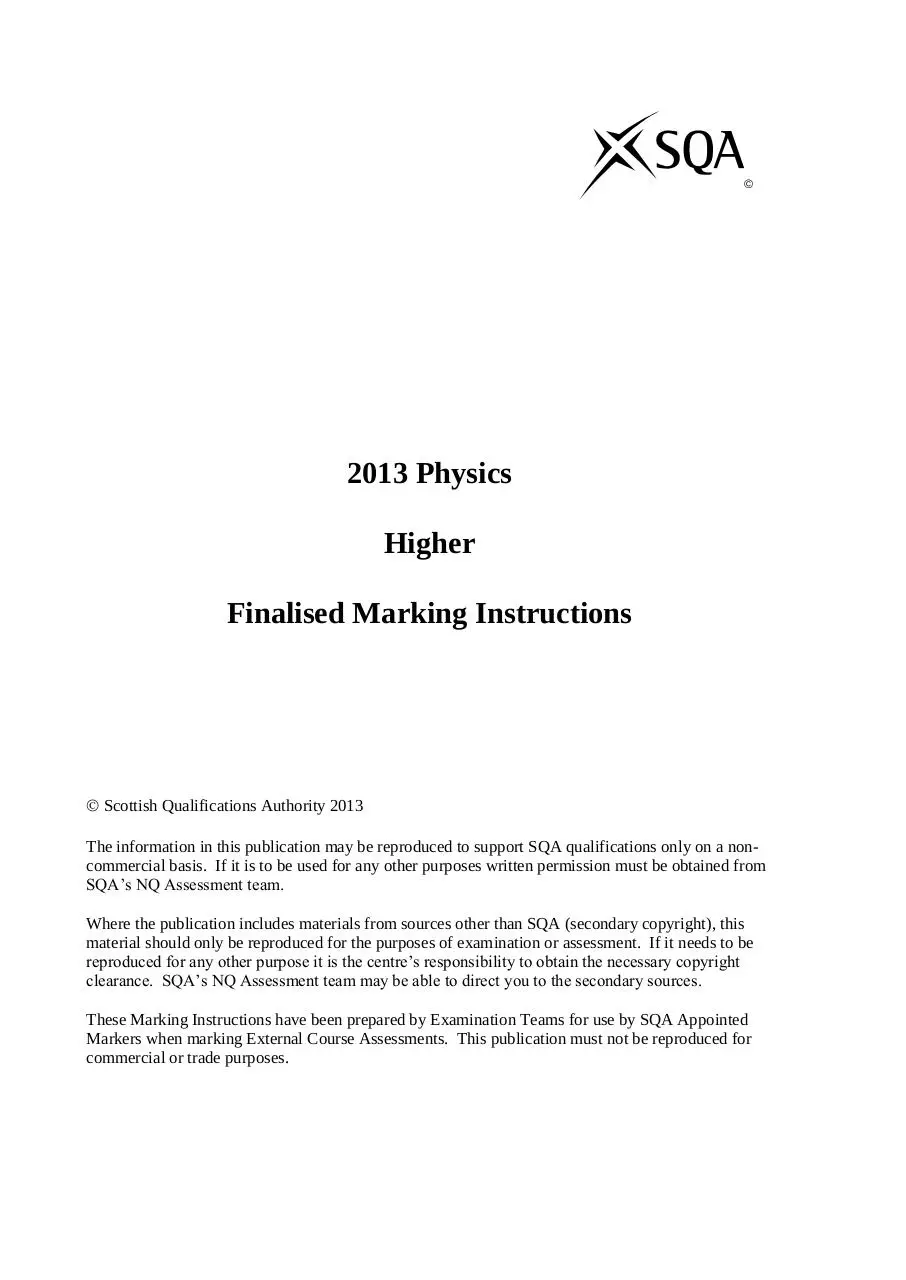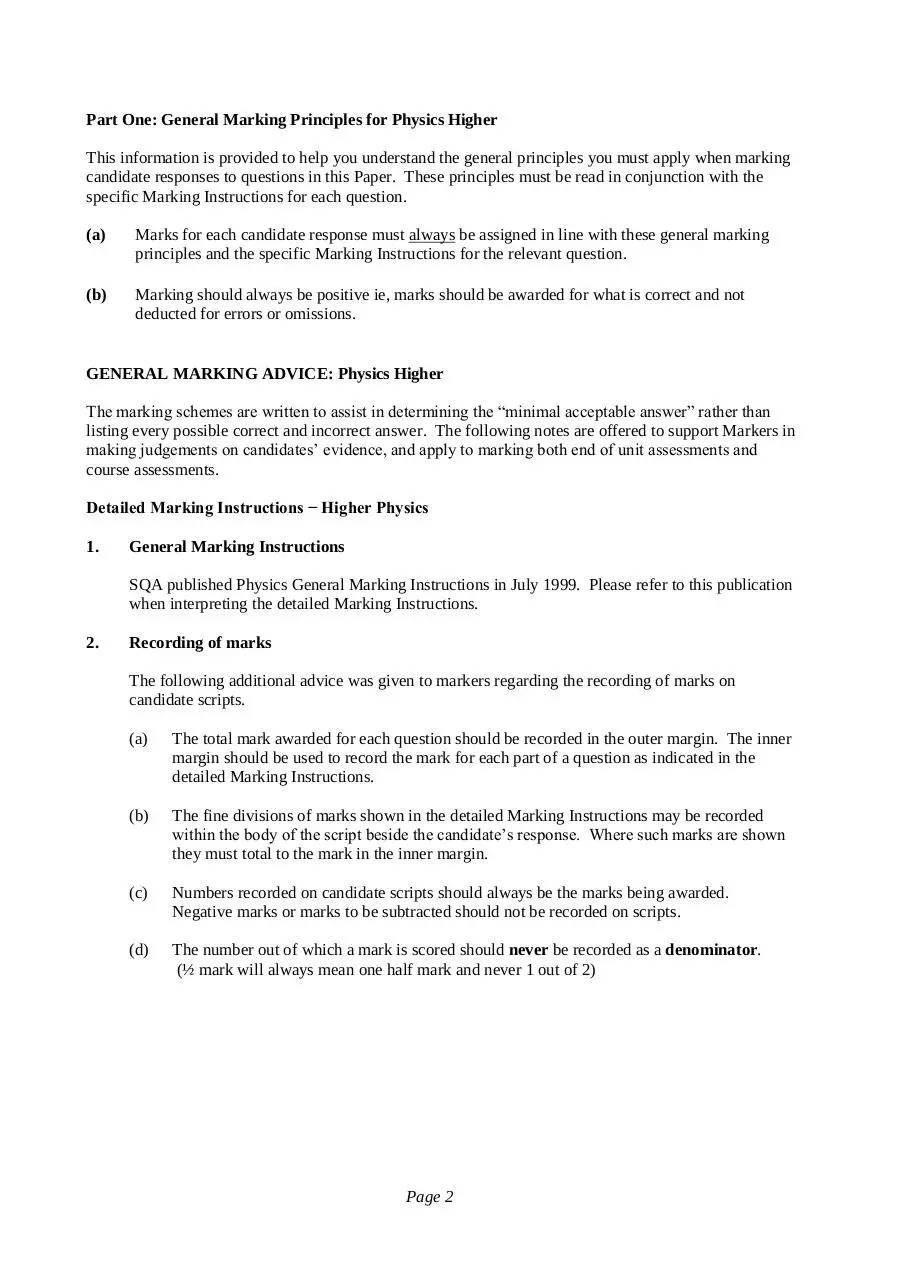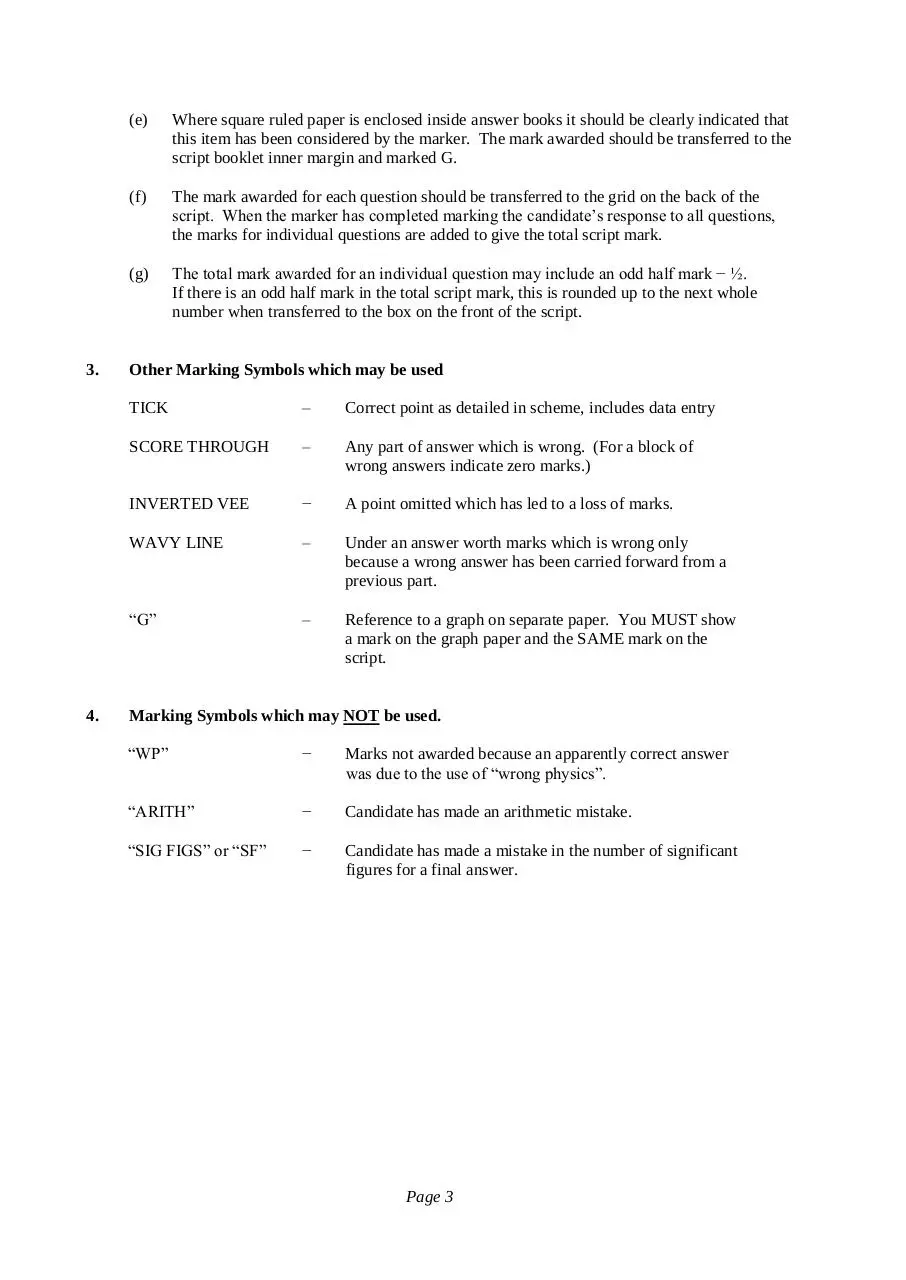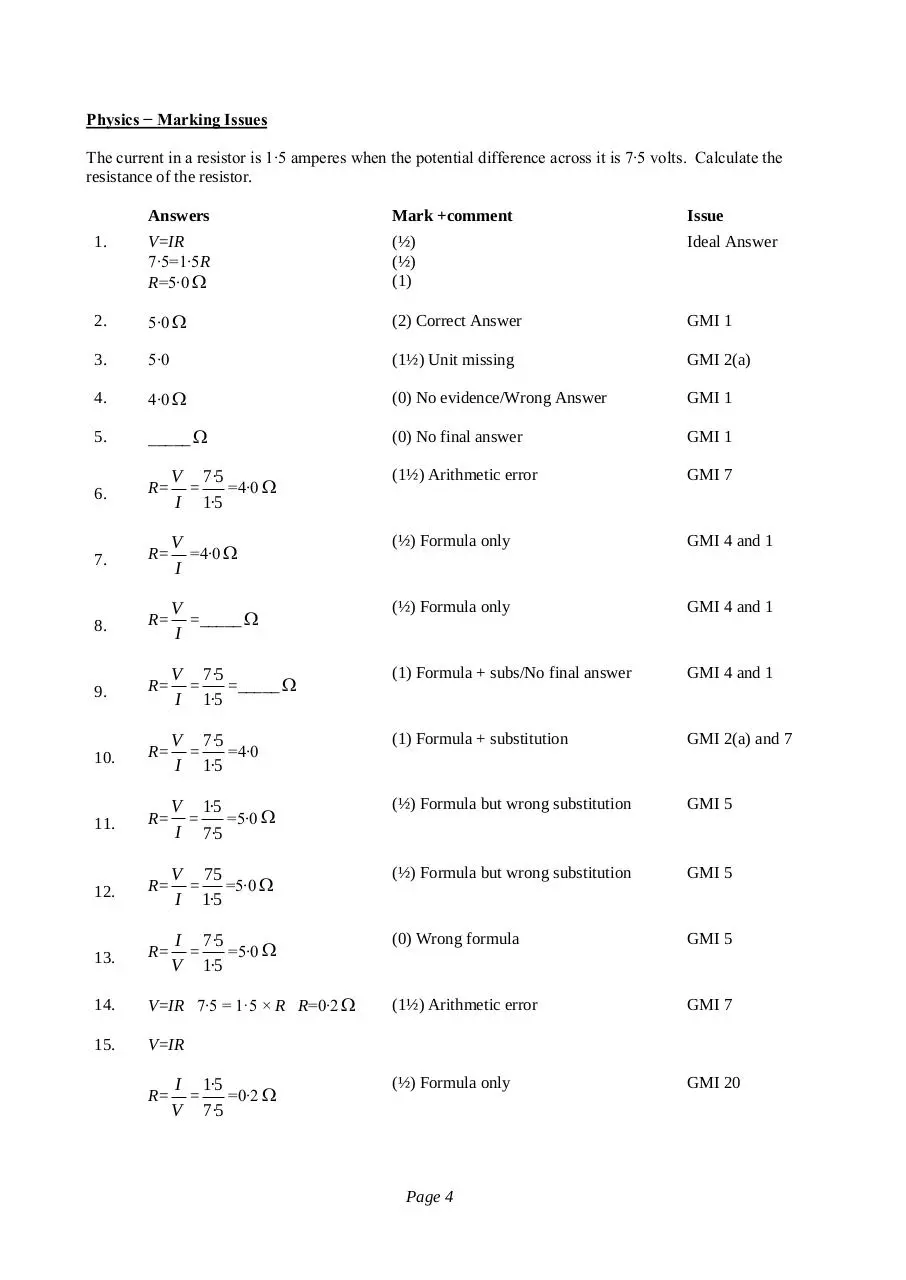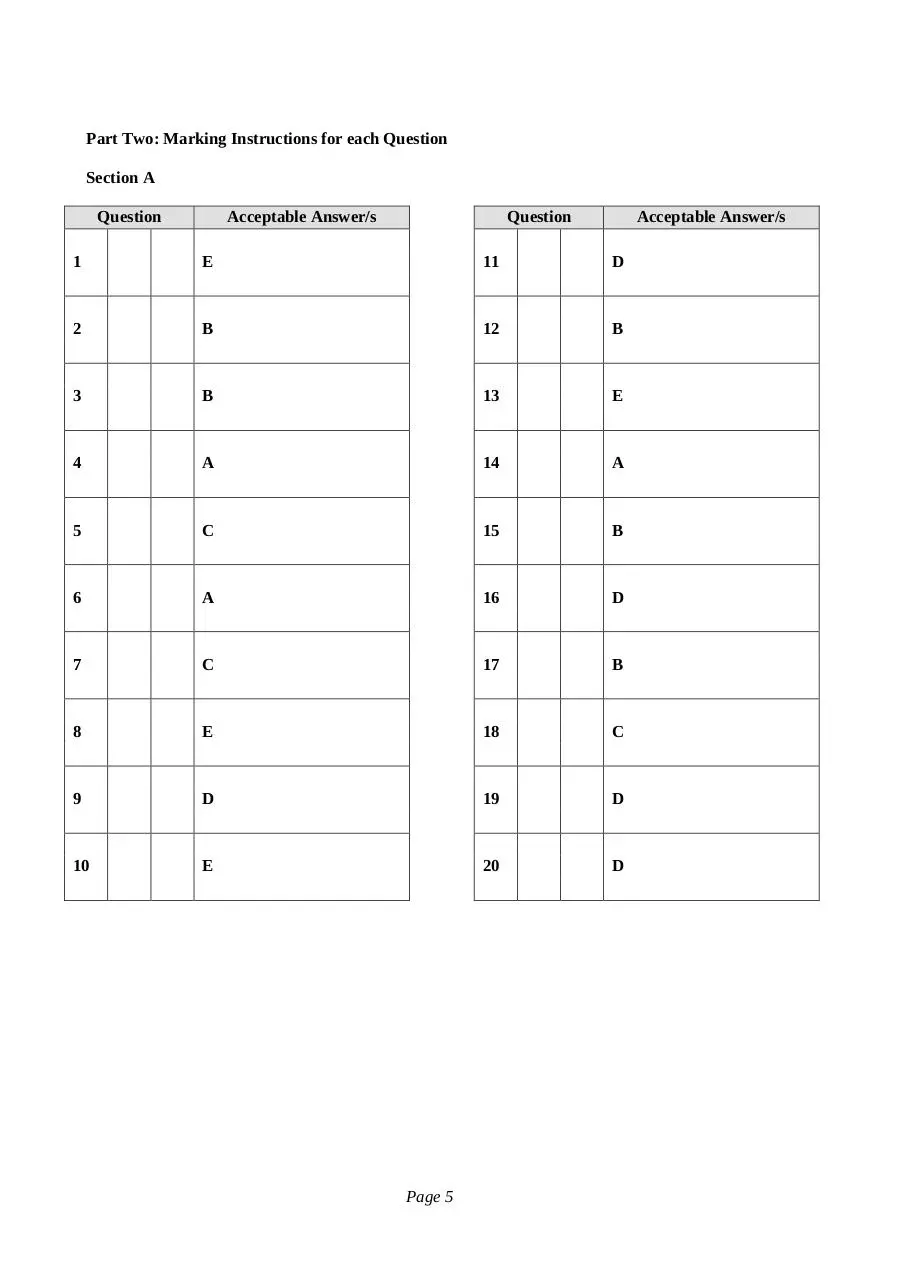mi_H_Physics_all_2013.pdf (PDF, 695.98 KB)

### Share on social networks

#### HTML Code

Copy the following HTML code to share your document on a Website or Blog